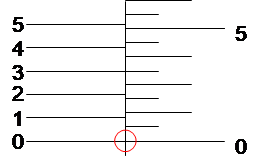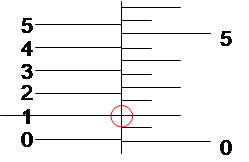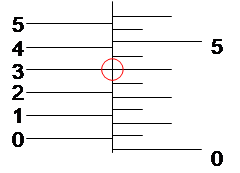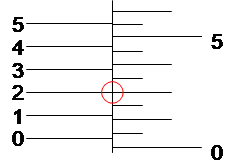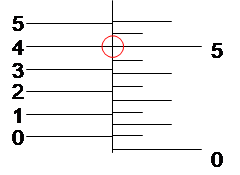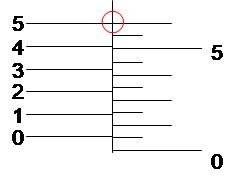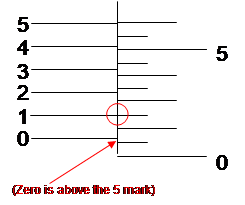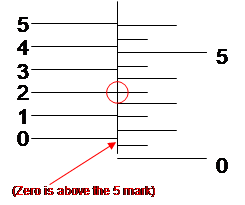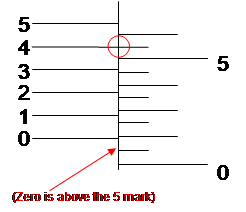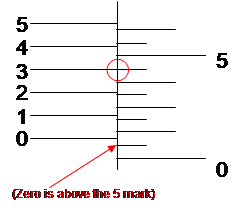# Using a Vernier Micrometer (Inch scale)

For rough reading (close thousandths), use the digital number.  However, it is likely not precise enough for precise thousandths or ten thousandths.  (You can see it seems to indicate about 0.0405, when actually, the marks on the right indicate 0.0410.)   I’ll show you what I mean as we proceed.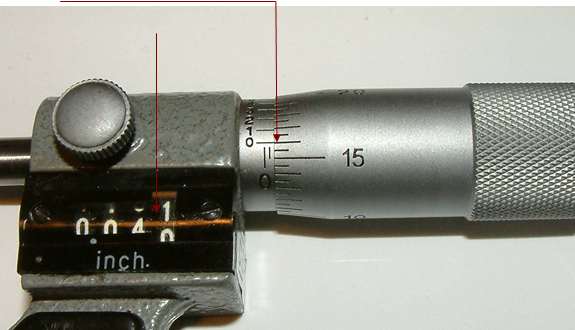For our purposes here, we’ll ignore the digital “odometer.”

## The Scales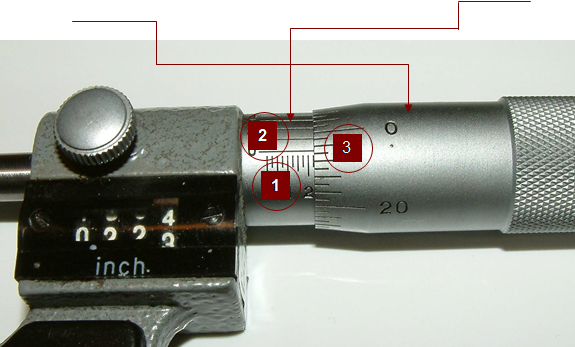The micrometer has three scales for measurement.  Two are on the sleeve, and one is on the thimble.  Together, they provide an accuracy of ten thousandths of an inch.

## Scale #1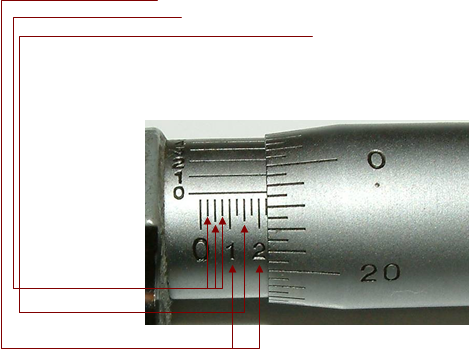The whole numbers on the horizontal scale on the sleeve measure tenths of an inch (0.0).  Each mark between these whole numbers measures twenty-five thousandths (0.025).  So the larger mark half way between the 1 and the 2 on the horizontal scale represents fifteen hundredths (0.15, the 1+ halfway to the 2); the first mark to the right of the 1 represents one hundred twenty-five thousandths (0.125); and the third mark to the right of the 1 represents one hundred seventy-five thousandths (0.175).

## Scale #2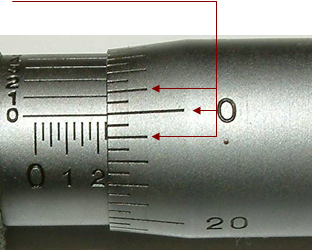On the thimble, the primary and secondary marks measure thousandths of an inch.  The measurement below is 0.225.

## Scale #3

On the sleeve, the vertical scale is used in conjunction with the vertical scale on the thimble to measure ten thousandths of an inch.  Here’s how it works: When the zero on the thimble aligns with the zero on the vertical sleeve scale, there are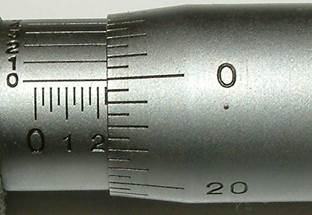Zero ten thousandths, as shown below.

So, this micrometer reading is 0.2250.  Next, we’ll go into how to read the ten thousandths place.

## Ten Thousandths

For precise ten thousandths, look at the horizontal gauge on the sleeve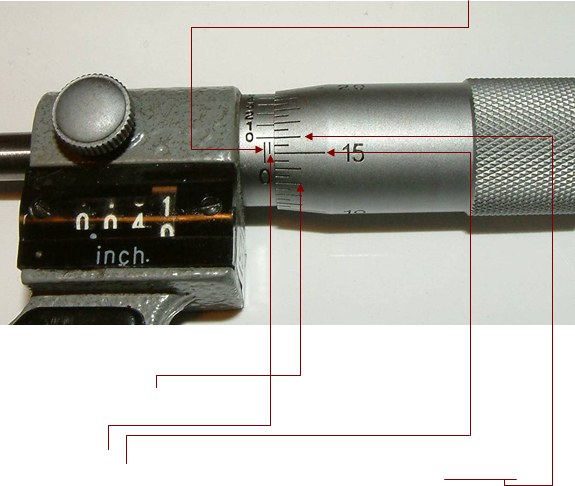Remember, on the horizontal sleeve scale, each mark above zero is 0.025 inch.

The marks on the thimble each represent 0.0005, so the 15 in the above photo represents 0.015; therefore each two marks represent 0.001 (one thousandth).

We see one mark above zero on the sleeve, so that reading is [0.025 on the sleeve gauge] + [0.015 +  (2 * 0.0005 = 0.001) on the thimble, which is 16] = a measurement of 0.025 + 16 = 0.041 or 41 thousandths of an inch.  Notice that the 16 mark (two marks above the 15) on the thimble.  It aligns with the zero on the sleeve’s vertical scale.  That means it is showing 0.0410, or 410 ten thousandths of an inch.  That is a clue to how to measure ten thousandths.  Keep reading.

To make measurements of ten thousandths, you implement the thimble and sleeve together.  Locate the mark on the thimble that lines with one of the marks on the sleeve.  The thimble mark that lines up most closely with one of the sleeve marks indicates which ten thousandth is indicated.  In the photograph below, the 2 on the sleeve lines up with a mark on the thimble, so we add 0.0002 to the 0.025 + 0.021 + 0.0002 = 0.0462.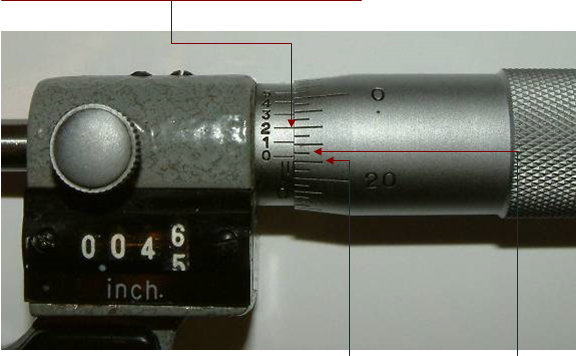Notice how the zero on the sleeve is above the 21 mark but below the 21.5 mark on the thimble?  That means the additional measurement will be less than 0.0005.  In this case, it’s 0.0002.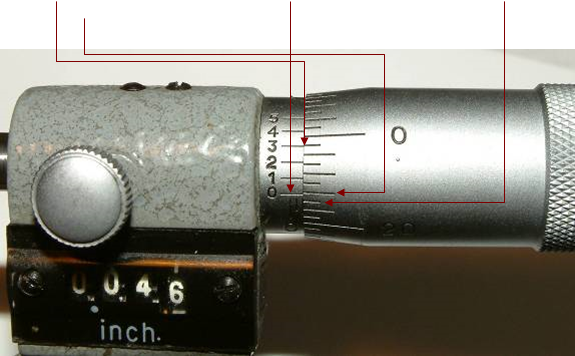Now, in the photograph below, you can see that the measurement lines up with the three.  However notice that the zero on the sleeve is above the 21.5 mark but below the 22 mark on the thimble.  That indicates the additional measurement will be greater than 0.0005.  In this case, it is 0.0008 instead of 0.0003 (5+3).

So the reading is 0.0468.  That is, 0.025 from the horizontal sleeve scale, + 0.0215 from the thimble scale, and 0.0003 from the vertical sleeve scale.

To recap, to read the ten thousandths, you find the line on the thimble that most closely aligns with a mark on the vertical scale of the sleeve.  If the zero on the sleeve’s vertical scale is below a “5” mark on the thimble (the shortest marks on the thimble), the number indicated on the sleeve is the ten thousandths value.  If the zero on the sleeve’s vertical scale is above a “5” mark on the thimble, add 5 to the number indicated on the sleeve to get the ten thousandths value.

Once again, the digital numbers to the left are irrelevant for measuring more precisely than rough thousandths.

Let’s consider a bit more closely how to read the ten thousandths.  I’ll demonstrate sequentially how it works and what it looks like.